Nisha Desai
7

Guide to ACT Math Geometry + Practice Questions

Do you know how to improve your profile for college applications?

See how your profile ranks among thousands of other students using CollegeVine. Calculate your chances at your dream schools and learn what areas you need to improve right now — it only takes 3 minutes and it's 100% free.

What’s Covered:

Geometry can be a difficult subject since it encompasses a wide variety of topics, including shapes, area and volume, angles, coordinates, and more! The ACT Math Test expects you to have a strong grasp of geometry, so you’ll need to be able to master all these concepts.

In this post, we’ll cover examples of some difficult types of geometry questions you may encounter on the exam with detailed solutions and strategies.

Overview of ACT Math Geometry

The ACT Math Test consists of 60 questions to be answered in 60 minutes. Each question is multiple choice and is weighted equally. A calculator is allowed for the entire math section, however, all the math questions are technically able to be solved without a calculator.

Geometry questions constitute 12–15% (or, about 7–9 questions) of the ACT Math Test. These questions test your ability to solve problems regarding topics like shapes, solids, area, volume, angles, and measurement.

Along with your overall score for the ACT Math Test, you’ll receive subscores for each subsection, including geometry. The ACT also provides the following directions for the math section of the exam: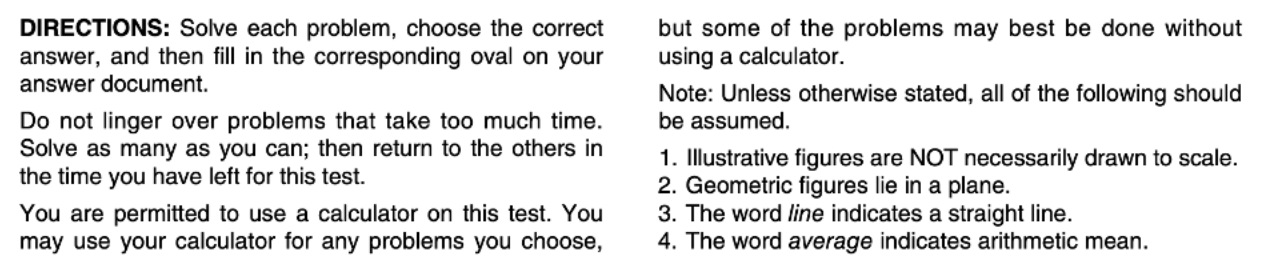We recommend familiarizing yourself with these directions prior to taking the exam to allow yourself more time on test day.

Also, the ACT Math Test doesn’t provide any formulas! This is especially important with the geometry questions, since you’ll need to have the formulas for area, perimeter, and volume of different shapes memorized. A comprehensive list of what you’ll need to know for the ACT Math test can be found in our article 31 ACT Math Formulas You Need to Know.

How Will the ACT Impact My College Chances?

Your ACT score is used by most colleges as an indicator of your academic strength. This means that scoring well on the ACT is necessary for admission into top colleges across the country. Some selective schools even automatically reject applicants with test scores and GPA that are below a certain threshold.

To see how your score stacks up against other applicants, try out CollegeVine’s free Admissions Calculator! This tool will analyze your test scores, GPA, course rigor, extracurriculars, and more to determine your chances at various schools. It will even point out where your admissions profile has room for improvement.10 Difficult Geometry Questions

Question 1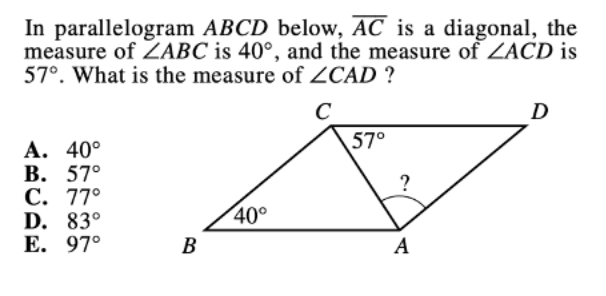This question requires the use of the Alternate Interior Angles Theorem, which states that alternate angles are congruent. The following diagram illustrates the alternate interior angles that we’ll be looking at:So, we’ll need to solve for x. The next thing we’ll need to recall is that the sum of the angles in a triangle is 180º. So, if we look at triangle ABC, we get the following equation:

57º+xº+40º = 180º

This means that x = 83, which corresponds to answer D.

Question 2As with most geometry problems, drawing a picture is a great way to start. Let’s draw a picture of the different walls in Laura’s room:The area formula for rectangles is A=bh, which means that we’ll need to multiply the base and height for each rectangle.

The first rectangle has area: A = 8 × 10 = 80.

The second rectangle has area: A = 8 × 15 = 120.

But, remember that Laura has two of each wall, so the total surface area of the walls is:

2(80) + 2(120) = 400

But, we have to subtract the area of the window and the door, since these won’t be painted.

Then, the answer is: 400 – 60 = 340 square feet.

Question 3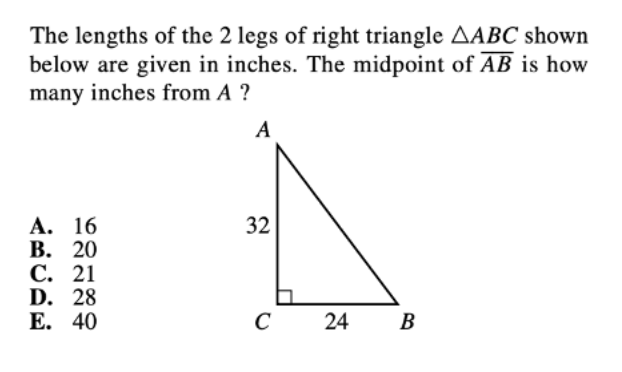To find the midpoint of AB, we’ll need to know its length. We can find its length using the Pythagorean Theorem.

For any right triangle, we have the classic formula: AC2 + BC2 = AB2.

We can solve for AB

322 + 242 = AB2

AB = 40

But, we’re not done here. The questions asks about the midpoint of AB, so we need to divide our length by 2. Then, our answer is: 40/2 = 20.

Question 4To solve this question, we’ll first need to isolate y:

4x = 7y + 5

7y = 4x – 5

y = 4/7x – 5/7

Since our equation is in the form y = mx + b (where m is the slope and b is the slope intercept), we have that the slope m = 4/7.

These types of questions can be easily missed due to minor calculation errors, so be sure to double check your work!

Question 5Let’s start by sketching out what we know: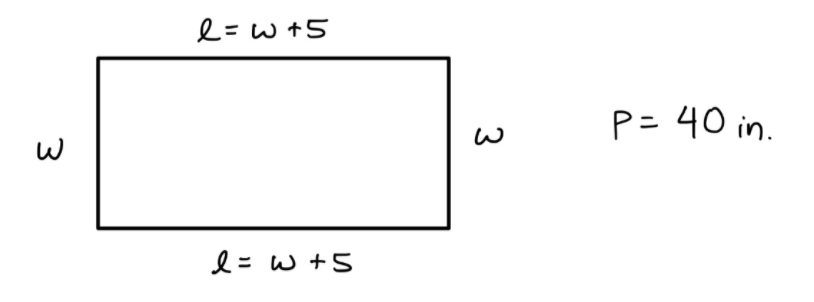Since we have that the perimeter of a rectangle is given by P = 2l + 2w, we can substitute the given information into the equation:

40 = 2(w+5) + 2w

40 = 2w + 10 + 2w

40 = 4w + 10

30 = 4w

w = 7.5 in

Question 6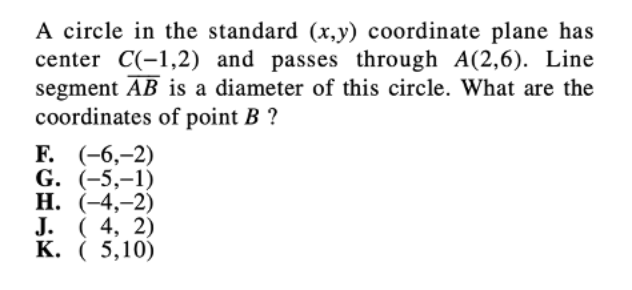Again, drawing a picture will help you to easily visualize the given information. We can start by plotting points A and C.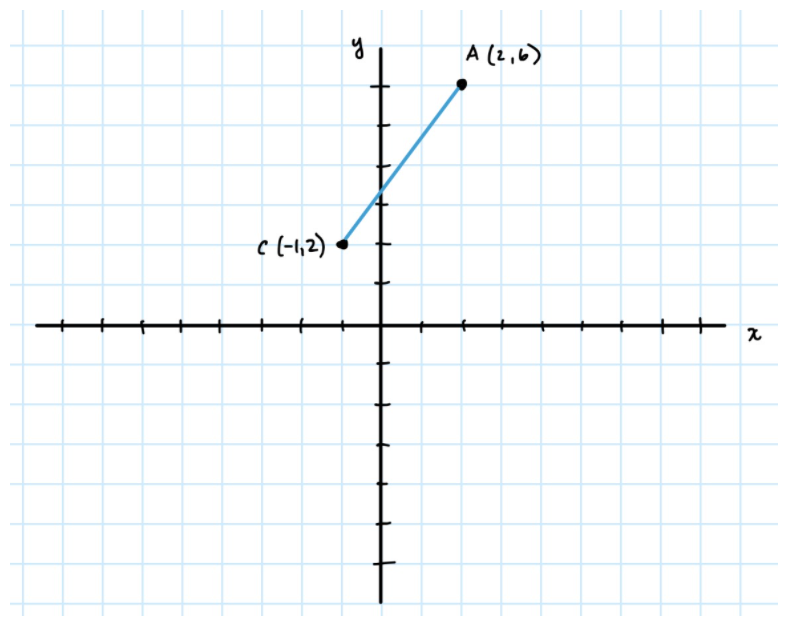Since AB is a diameter, it passes through the point C. This means that the slope from A to C should be equal to the slope from B to C (since all three points lie on the same line). We can use that information to construct where B would be:From the drawing, we see that we go up 4 units and right 3 units to get from C to A. So, to get from C to B, we can do the exact opposite. We go down 4 units and left 3 units to get that B is at (-4,-2).

Discover your chances at hundreds of schools

Our free chancing engine takes into account your history, background, test scores, and extracurricular activities to show you your real chances of admission—and how to improve them.

Question 7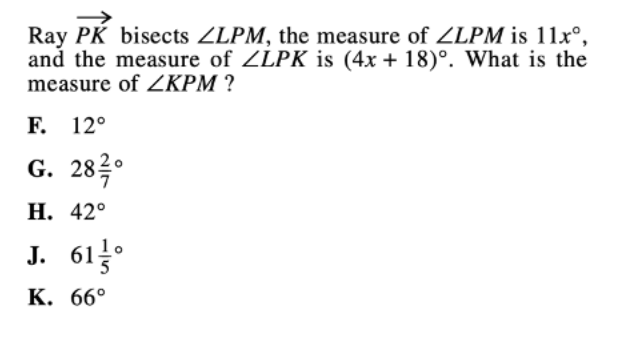Though this problem contains a lot of geometry terms, once you sort out the information, it’s mostly algebra. Remember that if the ray bisects the angle, it will form two congruent angles. Here is what that would look like:Since the ray bisects ∠LPM, we know that the measures of ∠LPK and ∠KPM are congruent. So, ∠KPM also has to measure 4x + 18º. To figure out the exact measure, we’ll need to solve for x.

Since ∠LPK and ∠KPM together make up ∠LPM, we get the following equation:

(4x+18) + (4x+18) = 11x

8x + 36 = 11x

3x = 36

x = 12

We can now use this value of x to find the measure of ∠KPM:

4(12) + 18 = 66º.

Question 8To determine the volume of the toy soldier, we’ll need to compare the volumes before and after the toy is submerged in water: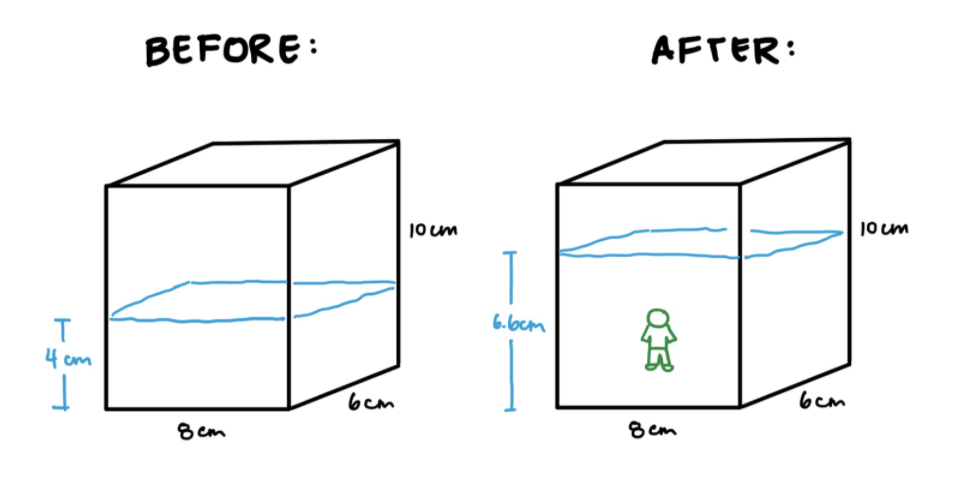You’ll have to memorize the formula for volumes of rectangular prisms: V=lwh, where l is the length, w is the width, and h is the height.

Then, the volume of the water before is: Vwater = 8 × 6 × 4 = 192 cm3

And, the volume of the water after the soldier is added is: Vsoldier and water = 8 × 6 × 6.6 = 316.8 cm3.

Finally, we can find the volume of the soldier by subtracting: 316.8 – 192 = 124.8125 cm3.

Question 9This problem is particularly difficult since it involves a lot of steps. First, we’ll need to figure out the perimeter of the park in Mikea’s scale drawing. But, we are missing the length of one side of the trapezoid, so we’ll have to use the Pythagorean theorem to find it.

Let’s start by constructing a line to form a right triangle:We already know that the height is 16 inches, so if we find x, we can use the Pythagorean Theorem to find the missing side length, y.

We can solve for x since we have the following equation:

x + 28 = 40

So, we get that x = 12.

Now, the Pythagorean Theorem guarantees that x2 + 162 = y2. If we plug in our new value for x, we get:

122 + 162 = y2 y = 20.

We can now find the perimeter of the trapezoid by adding all of our side lengths:

P = 20 + 28 + 16 + 40 = 104 in.

But, recall that our answer should be in feet. We were told that 1 inch represents 1.5 feet, so let’s convert our perimeter:

104 in × (1.5 feet/1 in) = 156 feet.

Question 10For clarity, I redrew the picture of the rectangular floor plan:Rather than directly compute the area of the walkway, we can simplify the problem by subtracting the area of the red rectangle from the area of the blue rectangle. Then, all that will be remaining is the walkway, which is what the question wants.

So, let’s compute the areas:

Ared = 24 × 30 = 720

Ablue =30 × 36 = 1080

Then, Awalkway = Ablue Ared = 1080 – 720 = 360 ft2.

Final Tips and Strategies

Draw a picture

As you’ve probably noticed with the preceding practice questions, drawing a picture makes geometry questions much easier. Since geometry deals with a lot of visual information (i.e. shapes and coordinates), it makes sense to draw out the information given.

Starting with a quick sketch will help you contextualize the given information and determine how best to solve the problem. Though your drawings don’t have to be to perfectly scale (after all these are meant to save you time, not take up more time), try to keep your sketches as accurate as possible. For example, if you’re drawing a 4×6 rectangle, it probably wouldn’t look like this:Keeping your drawings relatively accurate will help you determine if your final answer makes sense within the context of the problem. As you take practice tests, try drawing whenever possible so that you get into the habit of it.

Learn how to use your calculator

Because a calculator is allowed for the entire test, it’s a good idea to familiarize yourself with your calculator. If possible, try practicing with the same calculator that you’ll be using on test day. This will save you precious test-taking time and will even help you learn useful tricks and shortcuts to make the problems a little bit easier.

But, be wary of over-relying on your calculator. The ACT Math Test is designed so that a calculator is not technically necessary for any problem. So, even though you are allowed to use a calculator, that doesn’t necessarily mean that using a calculator is the most efficient way to solve a problem.

As you take practice tests, try repeating the same question with and without a calculator to see which method is the fastest and most accurate. This will help you realize which types of questions require the use of your calculator, maximizing your test-taking time.

Check out some of our other posts as you study for the ACT Math Test:Short Bio
Nisha Desai is a second year student at the University of California, Riverside. She recently started working at CollegeVine, but has done application guidance and tutoring in a private capacity for a couple of years. She is in school to eventually get her Masters in Education and enjoys reading and running in her free time.

Don't miss out on the best high school & college admissions resources!

Join thousands of students and parents getting exclusive high school, test prep, and college admissions information.